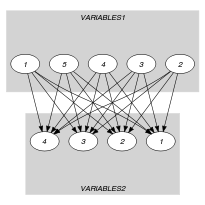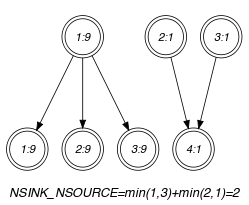## 5.367. soft_used_by_modulo_var

Origin
Constraint

$\mathrm{𝚜𝚘𝚏𝚝}_\mathrm{𝚞𝚜𝚎𝚍}_\mathrm{𝚋𝚢}_\mathrm{𝚖𝚘𝚍𝚞𝚕𝚘}_\mathrm{𝚟𝚊𝚛}\left(𝙲,\mathrm{𝚅𝙰𝚁𝙸𝙰𝙱𝙻𝙴𝚂}\mathtt{1},\mathrm{𝚅𝙰𝚁𝙸𝙰𝙱𝙻𝙴𝚂}\mathtt{2},𝙼\right)$

Synonym

$\mathrm{𝚜𝚘𝚏𝚝}_\mathrm{𝚞𝚜𝚎𝚍}_\mathrm{𝚋𝚢}_\mathrm{𝚖𝚘𝚍𝚞𝚕𝚘}$.

Arguments
 $𝙲$ $\mathrm{𝚍𝚟𝚊𝚛}$ $\mathrm{𝚅𝙰𝚁𝙸𝙰𝙱𝙻𝙴𝚂}\mathtt{1}$ $\mathrm{𝚌𝚘𝚕𝚕𝚎𝚌𝚝𝚒𝚘𝚗}\left(\mathrm{𝚟𝚊𝚛}-\mathrm{𝚍𝚟𝚊𝚛}\right)$ $\mathrm{𝚅𝙰𝚁𝙸𝙰𝙱𝙻𝙴𝚂}\mathtt{2}$ $\mathrm{𝚌𝚘𝚕𝚕𝚎𝚌𝚝𝚒𝚘𝚗}\left(\mathrm{𝚟𝚊𝚛}-\mathrm{𝚍𝚟𝚊𝚛}\right)$ $𝙼$ $\mathrm{𝚒𝚗𝚝}$
Restrictions
 $𝙲\ge 0$ $𝙲\le |\mathrm{𝚅𝙰𝚁𝙸𝙰𝙱𝙻𝙴𝚂}\mathtt{2}|$ $|\mathrm{𝚅𝙰𝚁𝙸𝙰𝙱𝙻𝙴𝚂}\mathtt{1}|\ge |\mathrm{𝚅𝙰𝚁𝙸𝙰𝙱𝙻𝙴𝚂}\mathtt{2}|$ $\mathrm{𝚛𝚎𝚚𝚞𝚒𝚛𝚎𝚍}$$\left(\mathrm{𝚅𝙰𝚁𝙸𝙰𝙱𝙻𝙴𝚂}\mathtt{1},\mathrm{𝚟𝚊𝚛}\right)$ $\mathrm{𝚛𝚎𝚚𝚞𝚒𝚛𝚎𝚍}$$\left(\mathrm{𝚅𝙰𝚁𝙸𝙰𝙱𝙻𝙴𝚂}\mathtt{2},\mathrm{𝚟𝚊𝚛}\right)$ $𝙼>0$
Purpose

For each integer $R$ in $\left[0,𝙼-1\right]$, let $𝑁{\mathit{1}}_{R}$ (respectively $𝑁{\mathit{2}}_{R}$) denote the number of variables of $\mathrm{𝚅𝙰𝚁𝙸𝙰𝙱𝙻𝙴𝚂}\mathtt{1}$ (respectively $\mathrm{𝚅𝙰𝚁𝙸𝙰𝙱𝙻𝙴𝚂}\mathtt{2}$) that have $R$ as a rest when divided by $𝙼$. $𝙲$ is the minimum number of values to change in the $\mathrm{𝚅𝙰𝚁𝙸𝙰𝙱𝙻𝙴𝚂}\mathtt{1}$ and $\mathrm{𝚅𝙰𝚁𝙸𝙰𝙱𝙻𝙴𝚂}\mathtt{2}$ collections so that for all $R$ in $\left[0,𝙼-1\right]$ we have $𝑁{\mathit{2}}_{R}>0⇒𝑁{\mathit{1}}_{R}\ge 𝑁{\mathit{2}}_{R}$.

Example
$\left(2,〈9,1,1,8,8〉,〈9,9,9,1〉,3\right)$

In the example, the values of the collections $〈9,1,1,8,8〉$ and $〈9,9,9,1〉$ are respectively associated with the equivalence classes $9\mathrm{mod}3=0$, $1\mathrm{mod}3=1$, $1\mathrm{mod}3=1$, $8\mathrm{mod}3=2$, $8\mathrm{mod}3=2$ and $9\mathrm{mod}3=0$, $9\mathrm{mod}3=0$, $9\mathrm{mod}3=0$, $1\mathrm{mod}3=1$. Since there is a correspondence between two pairs of equivalence classes we must unset at least $4-2$ items (4 is the number of items of the $\mathrm{𝚅𝙰𝚁𝙸𝙰𝙱𝙻𝙴𝚂}\mathtt{2}$ collection). Consequently, the $\mathrm{𝚜𝚘𝚏𝚝}_\mathrm{𝚞𝚜𝚎𝚍}_\mathrm{𝚋𝚢}_\mathrm{𝚖𝚘𝚍𝚞𝚕𝚘}_\mathrm{𝚟𝚊𝚛}$ constraint holds since its first argument $𝙲$ is set to $4-2$.

Typical
 $𝙲>0$ $|\mathrm{𝚅𝙰𝚁𝙸𝙰𝙱𝙻𝙴𝚂}\mathtt{1}|>1$ $|\mathrm{𝚅𝙰𝚁𝙸𝙰𝙱𝙻𝙴𝚂}\mathtt{2}|>1$ $\mathrm{𝚛𝚊𝚗𝚐𝚎}$$\left(\mathrm{𝚅𝙰𝚁𝙸𝙰𝙱𝙻𝙴𝚂}\mathtt{1}.\mathrm{𝚟𝚊𝚛}\right)>1$ $\mathrm{𝚛𝚊𝚗𝚐𝚎}$$\left(\mathrm{𝚅𝙰𝚁𝙸𝙰𝙱𝙻𝙴𝚂}\mathtt{2}.\mathrm{𝚟𝚊𝚛}\right)>1$ $𝙼>1$ $𝙼<$$\mathrm{𝚖𝚊𝚡𝚟𝚊𝚕}$$\left(\mathrm{𝚅𝙰𝚁𝙸𝙰𝙱𝙻𝙴𝚂}\mathtt{1}.\mathrm{𝚟𝚊𝚛}\right)$ $𝙼<$$\mathrm{𝚖𝚊𝚡𝚟𝚊𝚕}$$\left(\mathrm{𝚅𝙰𝚁𝙸𝙰𝙱𝙻𝙴𝚂}\mathtt{2}.\mathrm{𝚟𝚊𝚛}\right)$
Symmetries
• Items of $\mathrm{𝚅𝙰𝚁𝙸𝙰𝙱𝙻𝙴𝚂}\mathtt{1}$ are permutable.

• Items of $\mathrm{𝚅𝙰𝚁𝙸𝙰𝙱𝙻𝙴𝚂}\mathtt{2}$ are permutable.

• An occurrence of a value $u$ of $\mathrm{𝚅𝙰𝚁𝙸𝙰𝙱𝙻𝙴𝚂}\mathtt{1}.\mathrm{𝚟𝚊𝚛}$ can be replaced by any other value $v$ such that $v$ is congruent to $u$ modulo $𝙼$.

• An occurrence of a value $u$ of $\mathrm{𝚅𝙰𝚁𝙸𝙰𝙱𝙻𝙴𝚂}\mathtt{2}.\mathrm{𝚟𝚊𝚛}$ can be replaced by any other value $v$ such that $v$ is congruent to $u$ modulo $𝙼$.

Usage
Keywords
Arc input(s)

$\mathrm{𝚅𝙰𝚁𝙸𝙰𝙱𝙻𝙴𝚂}\mathtt{1}$ $\mathrm{𝚅𝙰𝚁𝙸𝙰𝙱𝙻𝙴𝚂}\mathtt{2}$

Arc generator
$\mathrm{𝑃𝑅𝑂𝐷𝑈𝐶𝑇}$$↦\mathrm{𝚌𝚘𝚕𝚕𝚎𝚌𝚝𝚒𝚘𝚗}\left(\mathrm{𝚟𝚊𝚛𝚒𝚊𝚋𝚕𝚎𝚜}\mathtt{1},\mathrm{𝚟𝚊𝚛𝚒𝚊𝚋𝚕𝚎𝚜}\mathtt{2}\right)$

Arc arity
Arc constraint(s)
$\mathrm{𝚟𝚊𝚛𝚒𝚊𝚋𝚕𝚎𝚜}\mathtt{1}.\mathrm{𝚟𝚊𝚛}\mathrm{mod}𝙼=\mathrm{𝚟𝚊𝚛𝚒𝚊𝚋𝚕𝚎𝚜}\mathtt{2}.\mathrm{𝚟𝚊𝚛}\mathrm{mod}𝙼$
Graph property(ies)
$\mathrm{𝐍𝐒𝐈𝐍𝐊}_\mathrm{𝐍𝐒𝐎𝐔𝐑𝐂𝐄}$$=|\mathrm{𝚅𝙰𝚁𝙸𝙰𝙱𝙻𝙴𝚂}\mathtt{2}|-𝙲$

Graph model

Parts (A) and (B) of Figure 5.367.1 respectively show the initial and final graph associated with the Example slot. Since we use the $\mathrm{𝐍𝐒𝐈𝐍𝐊}_\mathrm{𝐍𝐒𝐎𝐔𝐑𝐂𝐄}$ graph property, the source and sink vertices of the final graph are stressed with a double circle. The $\mathrm{𝚜𝚘𝚏𝚝}_\mathrm{𝚞𝚜𝚎𝚍}_\mathrm{𝚋𝚢}_\mathrm{𝚖𝚘𝚍𝚞𝚕𝚘}_\mathrm{𝚟𝚊𝚛}$ constraint holds since the cost 2 corresponds to the difference between the number of variables of $\mathrm{𝚅𝙰𝚁𝙸𝙰𝙱𝙻𝙴𝚂}\mathtt{2}$ and the sum over the different connected components of the minimum number of sources and sinks.

##### Figure 5.367.1. Initial and final graph of the $\mathrm{𝚜𝚘𝚏𝚝}_\mathrm{𝚞𝚜𝚎𝚍}_\mathrm{𝚋𝚢}_\mathrm{𝚖𝚘𝚍𝚞𝚕𝚘}_\mathrm{𝚟𝚊𝚛}$ constraint(a) (b)